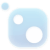# Top 20 NuGet function Packages

Data.HashFunction implementation of the Jenkins family of hash functions (http://en.wikipedia.org/wiki/Jenkins_hash_function). Note: SpookyHash can be found in the Data.HashFunction.SpookyHash package.
Data.HashFunction implementation of Fowler–Noll–Vo hash function (http://www.isthe.com/chongo/tech/comp/fnv/index.html) (http://en.wikipedia.org/wiki/FowlerâNollâVo_hash_function).
Data.HashFunction implementation of the hash function used by the elf64 object file format (http://downloads.openwatcom.org/ftp/devel/docs/elf-64-gen.pdf).
Data.HashFunction implementation of Bernstein hash (http://www.eternallyconfuzzled.com/tuts/algorithms/jsw_tut_hashing.aspx#djb).
Data.HashFunction implementation of the cyclic redundancy check (CRC) error-detecting code (http://en.wikipedia.org/wiki/Cyclic_redundancy_check). Implementation is generalized to encompass all possible CRC parameters from 1 to 64 bits.## The C++ Mathematical Expression Toolkit Library (ExprTk)

The C++ Mathematical Expression Toolkit Library (ExprTk) is a simple to use, easy to integrate and extremely efficient run-time mathematical expression parsing and evaluation engine. The parsing engine supports numerous forms of functional and logic processing semantics and is easily extendible.
The C++ Mathematical Expression Toolkit Library (ExprTk) Extra Examples
Generic Workflows as Action or Function
Data.HashFunction implementation of Bernstein hash (http://www.eternallyconfuzzled.com/tuts/algorithms/jsw_tut_hashing.aspx#djb).
Data.HashFunction implementation of BLAKE2 hash (http://blake2.net/).